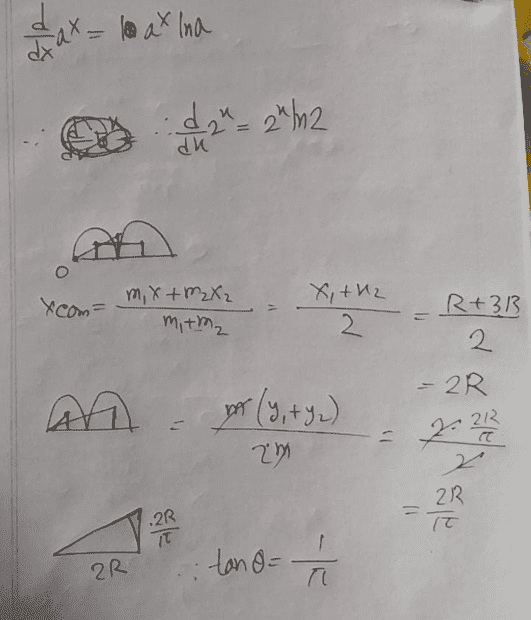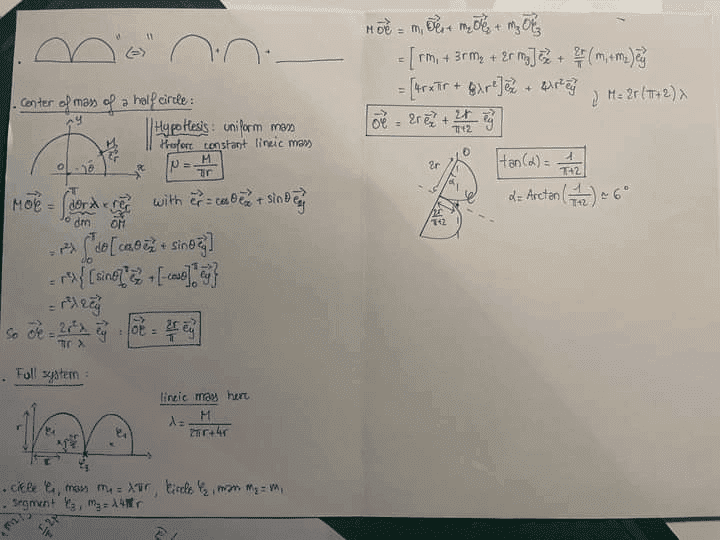# Find the center of mass of an arc

• Istiak
In summary, the conversation discusses the calculation of the center of mass of an arc in the shape of the letter "B". One person attempted to find the center of mass by treating the arc as a semicircle, but their answer was only an approximation. Another person solved the problem using a different method, but the picture they provided was blurry. The discrepancy between the two answers was attributed to the first solution not taking into account the mass of the upright line in the letter "B".

#### Istiak

Homework Statement
Find center of mass of a arc (letter B)
Relevant Equations
x_com = \frac{m_1 x_1 + m_2 x_2}{m_1+m_2}In my mind, I had cut half of B and, thought it's semi-circle. Then, I was trying to find Center of Mass by taking it as semi-circle. But, I get an answer which is approximately, close to main answer. Someone else had solved it another wayThis way I can get the accurate answer. But, the picture is very blurry that's why I can't understand it. Why both answers aren't same? Is there any other way to calculate Center of Mass?

Last edited:
Istiakshovon said:
Homework Statement:: Find center of mass of a arc (letter B)
Relevant Equations:: x_com = \frac{m_1 x_1 + m_2 x_2}{m_1+m_2}

View attachment 285438

In my mind, I had cut half of B and, thought it's semi-circle. Then, I was trying to find Center of Mass by taking it as semi-circle. But, I get an answer which is approximately, close to main answer. Someone else had solved it another way

View attachment 285439

This way I can get the accurate answer. But, the picture is very blurry that's why I can't understand it. Why both answers aren't same? Is there any other way to calculate Center of Mass?
Your solution seems to have ignored the mass of the upright line in "B".

•Delta2
haruspex said:
Your solution seems to have ignored the mass of the upright line in "B".
Which solution? I had attached two pictures. One is done by me (my friend) and, another had done by another person in Internet..

Yes your solution (first picture in the OP) doesn't take into account the "base line" of "B", that is the segment that consists of the diameters of the two semicircles and has total length 4r.

•Istiak